# NCERT Based Test: The Moving Coil Galvanometer

## 15 Questions MCQ Test Topic-wise MCQ Tests for NEET | NCERT Based Test: The Moving Coil Galvanometer

Description
Attempt NCERT Based Test: The Moving Coil Galvanometer | 15 questions in 15 minutes | Mock test for NEET preparation | Free important questions MCQ to study Topic-wise MCQ Tests for NEET for NEET Exam | Download free PDF with solutions
QUESTION: 1

### In a moving coil galvanometer the deflection (ϕ) on the scale by a pointer attached to the spring is

Solution:

Since magnetic torque on the coil,
τ = NIAB
This torque is balanced by counter torque
∴ kϕ =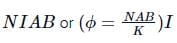where k is torsional constant. It is a scalar quantity having dimension of torque or energy i.e. [ML2T−2]

QUESTION: 2

### Two moving coil meters, M1 and M2 ave thhe following particulars: R1 = 10 Ω, N1 = 30, A1 = 3.6 × 10–3 m2, B1 = 0.25 T R2 = 14 Ω, N2 = 42, A2 = 1.8 × 10–3 m2, B2 = 0.50 T The spring constants are identical for the two meters. What is the ratio of  current sensitivity and voltage sensitivity of M2 and M1.

Solution:

For meter M1, R1 = 10 Ω, N1 = 30, A1 = 3.6 × 10–3 m2, B1 = 0.25 T, k= k
For meter M2, R2 = 14 Ω, N2 = 42, A2 = 1.8 × 10–3 m2, B2 = 0.50 T, k2 = k
As, current sensitivity Is = NBA/k.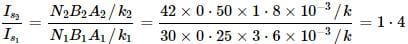Voltage sensitivity Vs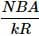So,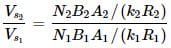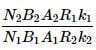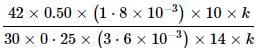= 1

QUESTION: 3

### If the current sensitivity of a galvanometer is doubled, then its voltage sensitivity will be

Solution:

Current sensitivity of galvanometer is deflection per unit current i.e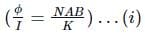Similarly voltage sensitivity is deflection per unit voltage
i.e.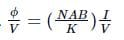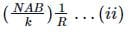From (i) and (ii)
Voltage sensitivity = current sensitivity × 1/resistance
Now if current sensitivity is doubled, then the resistance in the circuit will also be doubled since it is proportional to the length of the wire, then voltage sensitivity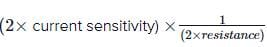= (current sensitivity) × 1 / (resistance)
Hence, voltage sensitivity will remain unchanged

QUESTION: 4

A moving coil galvanometer can be converted into an ammeter by:

Solution:

To utilise a galvanometer (G) as an ammeter, there is the difficulty in measurement of current due to the sensitivity of galvanometer and also the connection of galvanometer with a very large resistance in series that may change the value of current in the circuit.
To overcome these difficulties one attaches a small resistance rs called shunt resistance in parallel with the galvanometer coil as shown in the figure.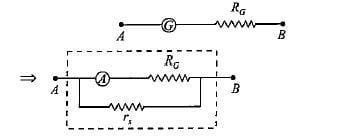QUESTION: 5

A galvanometer of resistance 70Ω, is converted to an ammeter by a shunt resistance rs = 0.03Ω. The value of its resistance will become

Solution: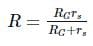Here, RG = 70Ω, rs = 0.03Ω
∴ R =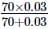= 0.02998
= 0.03Ω

QUESTION: 6

If the galvanometer current is 10mA, resistance of the galvanometer is 40Ω and shunt of 2Ω is connected to the galvanometer, the maximum current which can be measured by this ammeter is

Solution: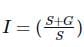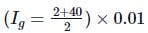= 0.21A

QUESTION: 7

A  galvanometer of resistance 40Ω gives a deflection of 5 divisions per mA. There are 50 divisions on the scale. The maximum current that can pass through it when a shunt resistance of 2Ω is connected is

Solution:

IG = 50/5 = 10mA; R= 40Ω, Rs = 2Ω
Maximum current,
I =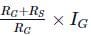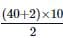= 210 mA

QUESTION: 8

In the given circuit, a galvanometer with a resistance of 70Ω is converted to an ammeter by a shunt resistance of 0.05Ω total current measured by this device is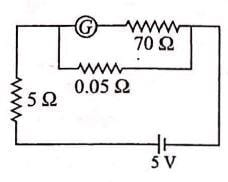Solution: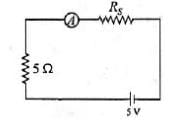The equivalent circuit of given circuit is given by
Here,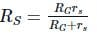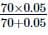∴ RS = 0.0499Ω = 0.05Ω
The total resistance in the circuit
R = RS + 5Ω = 0.05 + 5 = 5.05Ω
The current measured by the device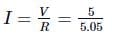= 0.99 A

QUESTION: 9

A galvanometer having a resistance of 50Ω, gives a full scale deflection for a current of 0.05A. The length (in metres) of a resistance wire of area of cross section 3 × 10−2cm2 that can be used to convert the galvanometer into an ammeter which can read a maximum of 5A current is
(Specific resistance of the wire ρ = 5 × 10−7Ωm)

Solution: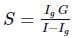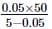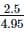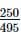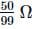∵ S =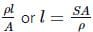∴ l  =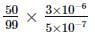= 3.0m

QUESTION: 10

The conversion of a moving coil galvanometer into a voltmeter is done by

Solution:

Galvanometer is a very sensitive instrument, therefore it can not measure high potential difference.
In order to convert a Galvanometer into voltmeter, a very high resistance known as "series resistance" is connected in series with the galvanometer.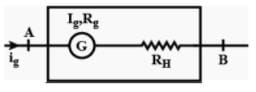QUESTION: 11

A galvanometer of resistance 10Ω gives full-scale deflection when 1mA current passes through it. The resistance required to convert it into a voltmeter reading upto 2.5V is

Solution:QUESTION: 12

A  voltmeter which can measure 2V is constructed by using a galvanometer of resistance 12Ω and that produces maximum deflection for the current of 2mA, then the resistance R is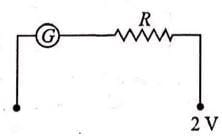Solution: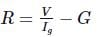Putting V = 2V,
Ig = 2mA
= 2 × 10−3A,
G = 12 Ω,
∴ R= 2 / 2 × 10−3 −12 = 1000 − 12

= 988Ω

QUESTION: 13

The value of current in the given circuit if the ammeter is a galvanometer with a resistance R= 50Ω is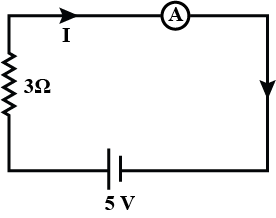Solution: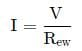Req = Rg +3 = 50 + 3 = 53Ω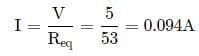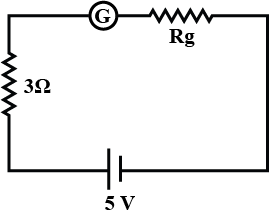QUESTION: 14

A galvanometer coil has a resistance of 15Ω and the metre shows full scale deflection for a current of 4mA. To convert the meter into a voltmeter of range 0 to 18V, the required resistance is

Solution:

The galvanometer can be transformed into a voltmeter by applying a high resistance in series. If the series resistance is R, then total resistance in circuit  = (15 + R)Ω
Now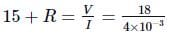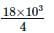= 4.5 × 10
∴ R  = 4500−15
= 4485Ω

QUESTION: 15

A galvanometer of resistance 50Ω is connected to a battery of 3V along with a resistance of 2950Ω in series. A full-scale deflection of 30 divisions is obtained in the galvanometer. In order to reduce this deflection to 20 divisions, the resistance in series should be:

Solution:

Total initial resistance
= G + R = 50Ω + 2950Ω = 3000Ω
Current,I =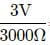= 1 × 10−3 A = 1mA
If the deflection has to be reduced to 20 divisions, then current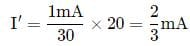Let x be the effective resistance of the circuit,
3V = 3000Ω × 1mA =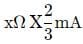or x =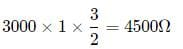∴  Resistance to be added =(4500Ω − 50Ω) = 4450ΩUse Code STAYHOME200 and get INR 200 additional OFF Use Coupon Code